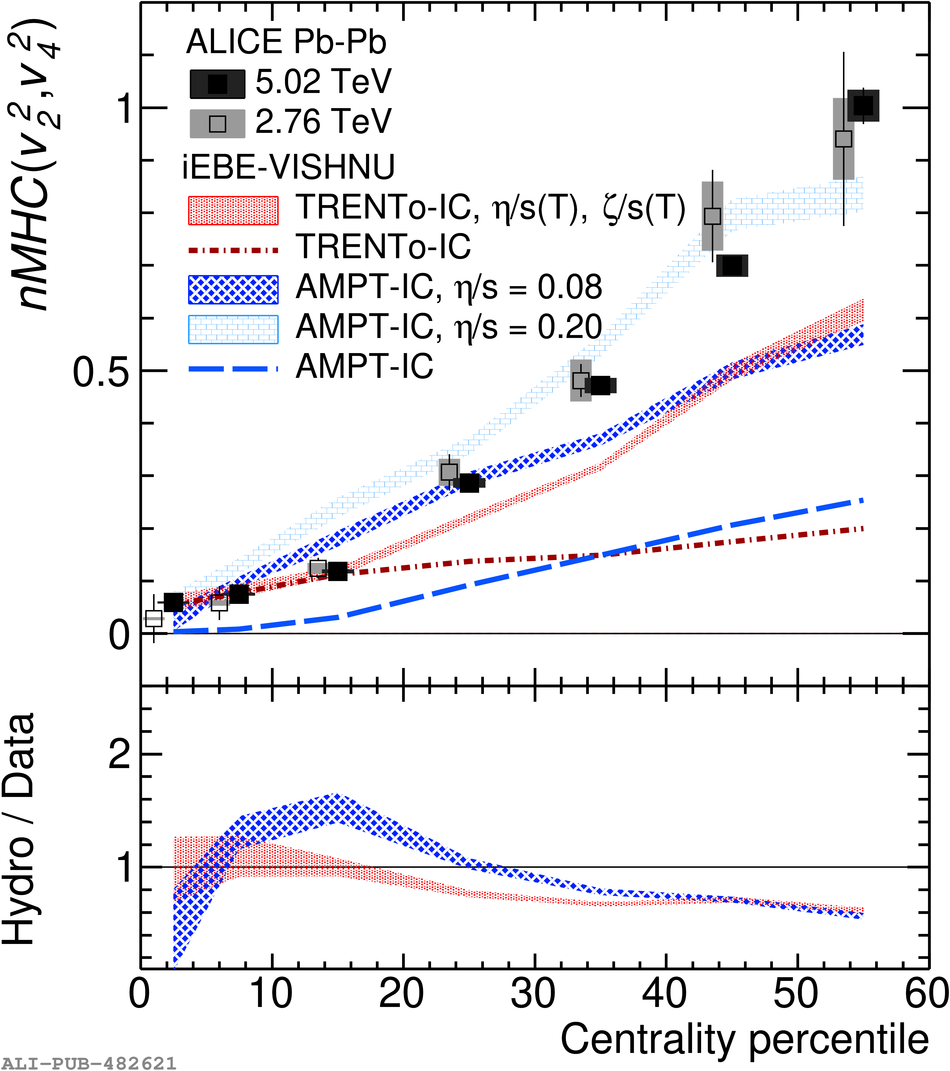# Figure 2

 < p>Centrality dependence of $nMHC(v_{2}^{2}, v_{3}^{2})$ and $nMHC(v_{2}^{2}, v_{4}^{2})$ in \PbPb\ collisions at $\sqrt{s_{\rm NN}} = 5.02$~TeV (solid markers) and 2.76 TeV (open markers). Statistical uncertainties are shown as vertical bars and systematic uncertainties as filled boxes. The iEBE-VISHNU calculations~ for Pb--Pb collisions at 5.02 TeV with TRENTo initial conditions (red shadowed bands) and AMPT initial conditions (blue shadowed bands) are presented, together with the corresponding initial state calculations $nMHC(\varepsilon_{2}^{2}, \varepsilon_{3}^{2})$, $nMHC(\varepsilon_{2}^{2}, \varepsilon_{4}^{2})$ from the TRENTo (red dot-dash lines) and AMPT model (blue long-dash lines). The same marker (line) styles and colors are used in later figures. Data points are shifted for visibility.< /p>